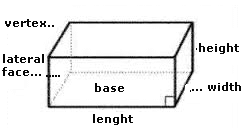### Rectangular prism

A rectangular prism is a three-dimensional solid figure with equal parallel edges, and two rectangles as base (top and bottom).
The rectangle prism has equal space diagonal and two different types of face diagonals.
Read full text in Prism.
Enter data using the point as a decimal separator. Ex 1,250.37 enter: 1250.37 , the results will be shown after a click on CalculateRectangular Prism Decimal places: Lenght: Width: Height: Total surface: Lateral surface: Area of the bases: Volume: Space diagonals: Lateral diagonal: Base diagonal: Front diagonal: Note:

Note:The accuracy of the calculator and its applicability to particular cases is not guaranteed.